#Who Remembers the Quadratic Equation?

Given a polynomial in which ax^2 + bx + c = 0, the quadratic equation, or “negative b plus or minus the square root of b squared minus 4 times a times c all divided by 2 times a” can solve for x. This formula has been seared in my brain since the 1980s.But now, apparently, there is a new way to solve for x that appeared in the most recent issue of Popular Mechanics.The images are from the Daily Mail today.

1. Arahant
@Arahant

Okay.

2. CB Toder aka Mama ToadWhat kills me is the idea that this is “easier.” Is it really? I never thought of the quadratic equation as hard to use…

3. Arahant
@Arahant

What kills me is the idea that this is “easier.” Is it really? I never thought of the quadratic equation as hard to use…

Bingo. But so easily derived now.

4. danok1
@danok1

What kills me is the idea that this is “easier.” Is it really? I never thought of the quadratic equation as hard to use…

Bingo. But so easily derived now.

Probably slightly easier to memorize as well.

5. DonG (skeptic)
@DonG

So, ‘a’ is not needed for the answer?   That is counter-intuitive.  I am skeptical.  That would mean these two equations have the same roots:

5x*x-6x-7 = 0     => (x= ??)
1x*x-6x-7 = 0      => (x=+7 or -1)

Somebody help me out.  It has been a long time.

A+ . . .

7. Kephalithos
@Kephalithos

What kills me is the idea that this is “easier.” Is it really? I never thought of the quadratic equation as hard to use…

Bingo. But so easily derived now.

The good people at Popular Mechanics seem to forget that most of us mortals inhabit a world of words and things, and not a world of numbers. We like formulas, and we don’t exactly cherish the thought of having to derive equations for ourselves.

8. PHCheese
@PHCheese

At least it’s easier than doing one’s taxes on the long form.

9. Barfly
@Barfly

So, ‘a’ is not needed for the answer? That is counter-intuitive. I am skeptical. That would mean these two equations have the same roots:

5x*x-6x-7 = 0 => (x= ??)
1x*x-6x-7 = 0 => (x=+7 or -1)

Somebody help me out. It has been a long time.

Soon as I get coffee. But you’re the best observer this morning – the correct solution does contain A.

10. CB Toder aka Mama ToadSo, ‘a’ is not needed for the answer? That is counter-intuitive. I am skeptical. That would mean these two equations have the same roots:

5x*x-6x-7 = 0 => (x= ??)
1x*x-6x-7 = 0 => (x=+7 or -1)

Somebody help me out. It has been a long time.

Solving your first equation with the quadratic equation, I get “3 plus or minus 4 times radical 11 all divided by 5,” but using this new formula I get 7 or -1, just as you do.

Maybe this is new math, but in my book 7 is not equal to 3 plus 4 times radical 11 all divided by 5… I’m confused.

Maybe this is just fake news?

11. Barfly
@Barfly

The error lies in the step that reads

… where -B = (R + S) and C = RS

The author reasoned those equivalences by matching the powers of x on each side, which is a fine idea, but he ignores the x-squared terms. That leaves us with assuming A equals 1.

The reasoning from that point forward is therefore incorrect. There wouldn’t be a problem here if the original problem statement hadn’t had that pesky A, but it does.

I see what he’s trying to do, but if one makes the necessary correction to the “simplified” derivation I think the algebra becomes more unwieldy, not less. I’ll stick with completing the square.

12. Barfly
@Barfly

I suppose we can patch it up by dividing the original equation thru by A, so that the x-squared term’s coefficient becomes 1, the x-term’s becomes B/A and the constant term’s coefficient becomes C/A. One may substitute those into the (erroneous) conclusion

x = -B/2 +/- ((B^2 / 4) -C)^1/2

which becomes a messy thing I can’t type here but rearranges easily to

x = [-B +/- (B^2 – 4AC)^1/2] / 2A

which is correct.

Ok, I’ll buy it – even with the late fix it’s arguably no harder than completing the square.

Somebody check my work, it’s really nice outside and I’m going to get a walk in this morning.

13. WillowSpring
@WillowSpringI remember the equation, but can’t remember any time after school that I used it.

In fact, in spite of a career as an Electrical Engineer, I only used Calculus once.  I was consulting on a project to control viewer that handled reel to reel photogrammetric film.  (The actual machine I worked on had been a prop in one of the early Ryan movies).

The goal was to select a specific footage and have the system run the film to that spot and stop.  It would have been trivial if there had been a footage counter, but that wasn’t available.  Instead, there was a revolution counter on the takeup spool.  The problem is that as the film is loaded onto spool, the effective diameter gets slightly larger, so the next revolution will pull slightly more film.  So enter Calculus.  I finally got it so it would get ‘close’ which wound up as ‘good enough’.

In spite of rarely using either, I am glad of having learned them.  In both cases, the approach shows you how to attack a problem.

14. Dr. Bastiat
@drbastiat

Wow.  That’s pretty cool…

15. Barfly
@Barfly

I goofed a little, I see after my walk. The error in the simplified derivation actually begins when it asserts that one may write

Ax^2 + Bx + C = (x – R)(x – S)

That is false. One may, however, write

Ax^2 + Bx + C = A(x – R)(x – S)

The logic of the derivation will then yield the correct quadratic formula.

16. Barfly
@Barfly

A+ . . .

@stad saw it from the beginning, of course, and has just been sitting back watching us.

I do not see how this is better than than the quadratic formula.    In any way.

18. Arahant
@Arahant

I do not see how this is better than than the quadratic formula. In any way.

20/20 vision.

19. Barfly
@Barfly

I do not see how this is better than than the quadratic formula. In any way.

It is the quadratic formula. Just an unusual derivation of it.

20. RightAngles
@RightAngles

I totally understood every word of this.

21. Clifford A. Brown
@CliffordBrown

At least it’s easier than doing one’s taxes on the long form.

With a stubby pencil and no eraser.

Anyone remember this fallacious proof?  Figure out what’s wrong (copied from the web):

# 1=2: A Proof using Beginning Algebra

The Fallacious Proof:

• Step 1: Let a=b.
• Step 2: Then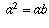,
• Step 3: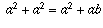,
• Step 4: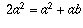,
• Step 5: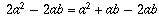,
• Step 6: and.
• Step 7: This can be written as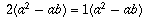,
• Step 8: and cancelling thefrom both sides gives 1=2.
23. Manny
@Manny

Surprisingly in this past year I had to use the quadratic equation for something at work.  (I’m an engineer.)  So I did have to refresh my memory of it.  But it is surprising how little mathematics I have had to use in my 34 years as an engineer.  The emphasis on math for engineering curriculum at college is skewed too much.  You have to understand the math behind the physics, but you rarely ever have to use math at work.  Anyway, that’s my thirty second vent for the day.

24. Manny
@Manny

Anyone remember this fallacious proof? Figure out what’s wrong (copied from the web):

# 1=2: A Proof using Beginning Algebra

The Fallacious Proof:

• Step 1: Let a=b.
• Step 2: Then,
• Step 3:,
• Step 4:,
• Step 5:,
• Step 6: and.
• Step 7: This can be written as,
• Step 8: and cancelling thefrom both sides gives 1=2.

That’s hilarious!

25. Manny
@Manny

I remember the equation, but can’t remember any time after school that I used it.

In fact, in spite of a career as an Electrical Engineer, I only used Calculus once. I was consulting on a project to control viewer that handled reel to reel photogrammetric film. (The actual machine I worked on had been a prop in one of the early Ryan movies).

Exactly.  I’ve rarely ever had to use math.  The same for me.  See above.

I totally understood every word of this.

Things like that happen when you’re a genius . . .

Anyone remember this fallacious proof? Figure out what’s wrong (copied from the web):

# 1=2: A Proof using Beginning Algebra

The Fallacious Proof:

• Step 1: Let a=b.
• Step 2: Then,
• Step 3:,
• Step 4:,
• Step 5:,
• Step 6: and.
• Step 7: This can be written as,
• Step 8: and cancelling thefrom both sides gives 1=2.

That’s hilarious!

The error (or fallacy if you prefer) in this “proof” is subtle . . .

28. Jerry Giordano (Arizona Patrio…
@ArizonaPatriot

Anyone remember this fallacious proof? Figure out what’s wrong (copied from the web):

# 1=2: A Proof using Beginning Algebra

The Fallacious Proof:

• Step 1: Let a=b.
• Step 2: Then,
• Step 3:,
• Step 4:,
• Step 5:,
• Step 6: and.
• Step 7: This can be written as,
• Step 8: and cancelling thefrom both sides gives 1=2.

For those who haven’t figured it out — “cancelling” the (a^2-ab) figure is dividing by zero, since a=b.  You can’t divide by zero.

29. Barfly
@Barfly

Anyone remember this fallacious proof? Figure out what’s wrong (copied from the web):

# 1=2: A Proof using Beginning Algebra

The Fallacious Proof:

• Step 1: Let a=b.
• Step 2: Then,
• Step 3:,
• Step 4:,
• Step 5:,
• Step 6: and.
• Step 7: This can be written as,
• Step 8: and cancelling thefrom both sides gives 1=2.

Ok, got it, but I’m not going to call it out unless I get a cookie.

30. Jerry Giordano (Arizona Patrio…
@ArizonaPatriot

I goofed a little, I see after my walk. The error in the simplified derivation actually begins when it asserts that one may write

Ax^2 + Bx + C = (x – R)(x – S)

That is false. One may, however, write

Ax^2 + Bx + C = A(x – R)(x – S)

The logic of the derivation will then yield the correct quadratic formula.

Yep, you nailed it, Barfly.

The formula presented erroneously assumes that A=1 (or a=1, depending on whether or not we are using capital or lower-case letters).

Of course, you can always force an equation into a form such that A=1.  If you start with ax^2 + bx = c = o, you can divide by a and redefine terms such that B=b/a and C=c/a, which then yields x^2 + Bx + C = 0.  In this case, the supposedly new formula is simply an algebraic transformation of the traditional quadratic formula for the special case in which a=1.

The Popular Mechanics article, and the video embedded therein, does not include the mistake stated in the OP that was identified by Barfly.  The video actually assumes that the equation is in the form x^2 + Bx + C = 0.